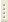06.02.2023X-Ray Structural Analysis
Prof. V.Sh.Shekhtman

The course introduces the basic principles of diffraction analysis of crystal atomic structure and the basic concepts of state-of-the-art atomic crystallography. Specific features of point and spatial groups, methods of symmetry characterization and physics of x-ray, electron and slow neutron-matter interaction are considered. Main experimental problems of diffraction study of atomic crystal structure, real structure and structural processes upon phase transformation are analyzed. Special emphasis is placed on recently determined structural states: quasicrystals, modulated structures, fullerenes.

Curriculum

1. Elements of crystallography. Spatial lattice theory.
1. Nodal straight line. Crystallographic plane. Elementary cell. Lattice constants. Types of symmetry transformations. Closed symmetry elements.
2. Combinations of symmetry elements. Generation of symmetry elements. Crystallographic point groups. Crystallographic systems: crystal systems. Matrix representation of symmetry operations and point groups. Curie limiting groups. Point groups of icosahedral crystal systems. Quasicrystal concept.
3. Translational groups. Elementary cell and 14 Bravais lattices choice laws. Crystal lattice-crystal atomic structure correlation.
4. Translation as symmetry element. Screw axes, glide-reflection planes. Matrix representation of open symmetry elements. International symmetry group notations.
5. Right systems of points, point orbits. General and partial equivalent position multiplicity. Rational arrangement and cell atom number in accordance with position multiplicity. Complete description of known crystal structure. Closest sphere packing. Stacking faults. Tetra- and octo- structural pores.
6.  Crystallographic polyhedrons. Simple shapes – plane orbits. Platon perfect forms as simple shapes of cubic and icosahedral crystal systems. Lattice and lattice complex. Crystallographic projection types: linear, gnomonic, stereographic, gnomostereographic. Wulf net.
7. Reciprocal space metric. Reciprocal lattice and related atomic crystal lattice.
2. X-ray production and X-ray-matter interaction.
1. X-ray units. X-ray tube design types.
2. X-ray spectrum.
3. Synchrotron radiation, physical principles of its production, research and technological applications.
4.  X-ray absorption and scattering in matter. Linear absorption factors, their wavelength and atomic number dependence. Selective absorption and filters.
5.  Free electron scattering. Thomson formula. Atomic scattering function. Derivation of general expression, methods of atomic scattering amplitude calculation.
3. X-ray crystal diffraction.
1. “Geometrical” theory of diffraction by three-dimensional scattering center grating. Wulf-Bragg equation in vector and scalar expression. Ewald structure. Brillouin zone boundary.
2. Main diffraction schemes in reciprocal lattice representation: Laue method, powder method, rotation (swing) method, wide diverging beam method, diffractometry of polycrystals and single crystals.
3.  X-ray reflection intensity. Single elementary cell scattering. Structural amplitude, derivation of general expression.
4.  Interference Laue function. Small crystal scattering problem. Conditions of appearance of diffraction maxima. Shape and size of reciprocal lattice sites.
5.  Transformation to working intensity equations. Specific plane system reflectivity. Temperature factor. Multiplicity factor. Angle factor.
6.  Structural amplitude analysis. Systematic X-ray reflection extinction related to Bravais lattice type, screw axis, glide-reflection planes.
7.  Fourier synthesis as a method of crystal atomic structure analysis. Three-dimensional Fourier expansion of electron density, structural amplitudes as series coefficients. Reciprocal lattice, site volume, geometric image of Fourier expansion.
4. Main problems solved by X-ray structural analysis.
1. Stages of unknown structure analysis. Sequence of application of different types of setting for determination of crystal systems, Bravais lattice type, point and spatial groups, number of atoms in elementary cell. Experimental and calculation methods of determination of atom position in cell. Trial-and-error method. Paterson synthesis, Fourier synthesis.
2. Problems of metal system analysis. Identification of phase regions on state diagrams. Solid solution ordering. Dispersion alloy hardening, “two-dimensional” nuclei related to duralumin aging.
3.  Experimental problems in studies of structural transformation and aperiodic crystals: diffusionless martensite transformations, polytypic crystals, modulated incommensurate structures.
5. Fundamentals of electron and neutron diffraction analysis
1. Electron diffraction analysis. Principles and specifics of high-energy electron diffraction. Application fields. Main problems for low-energy electron diffraction.
2. Neutron diffraction analysis. Nuclear scattering – application principles. Magnetic scattering, determination of ordering of magnetic moments in ferromagnetic and antiferromagnetic structures.
6. X-ray diffraction microscopy.
1. Kinematic and dynamic approximation, diffraction theories. Primary and secondary extinction. Perfect mosaic crystal.
2. Analysis of crystal real structure. Berg-Barrett method. Fujiwara, Shultz, angular scanning methods.
3. Methods of investigation of perfect crystals, application of phenomena in context of dynamic theory. Lang arrangement. Abnormal transmission effect, Borrmann method.